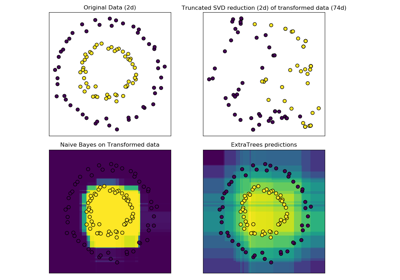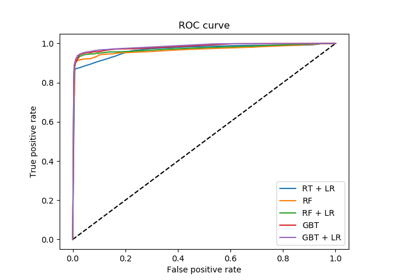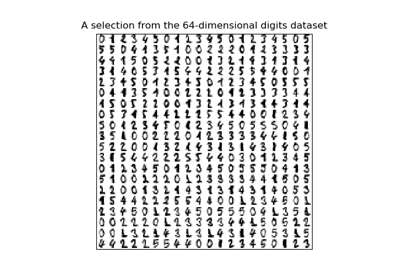# sklearn.ensemble.RandomTreesEmbedding¶

class sklearn.ensemble.RandomTreesEmbedding(n_estimators=100, max_depth=5, min_samples_split=2, min_samples_leaf=1, min_weight_fraction_leaf=0.0, max_leaf_nodes=None, min_impurity_decrease=0.0, min_impurity_split=None, sparse_output=True, n_jobs=None, random_state=None, verbose=0, warm_start=False)[source]

An ensemble of totally random trees.

An unsupervised transformation of a dataset to a high-dimensional sparse representation. A datapoint is coded according to which leaf of each tree it is sorted into. Using a one-hot encoding of the leaves, this leads to a binary coding with as many ones as there are trees in the forest.

The dimensionality of the resulting representation is n_out <= n_estimators * max_leaf_nodes. If max_leaf_nodes == None, the number of leaf nodes is at most n_estimators * 2 ** max_depth.

Read more in the User Guide.

Parameters
n_estimatorsinteger, optional (default=10)

Number of trees in the forest.

Changed in version 0.22: The default value of n_estimators changed from 10 to 100 in 0.22.

max_depthinteger, optional (default=5)

The maximum depth of each tree. If None, then nodes are expanded until all leaves are pure or until all leaves contain less than min_samples_split samples.

min_samples_splitint, float, optional (default=2)

The minimum number of samples required to split an internal node:

• If int, then consider min_samples_split as the minimum number.

• If float, then min_samples_split is a fraction and ceil(min_samples_split * n_samples) is the minimum number of samples for each split.

Changed in version 0.18: Added float values for fractions.

min_samples_leafint, float, optional (default=1)

The minimum number of samples required to be at a leaf node. A split point at any depth will only be considered if it leaves at least min_samples_leaf training samples in each of the left and right branches. This may have the effect of smoothing the model, especially in regression.

• If int, then consider min_samples_leaf as the minimum number.

• If float, then min_samples_leaf is a fraction and ceil(min_samples_leaf * n_samples) is the minimum number of samples for each node.

Changed in version 0.18: Added float values for fractions.

min_weight_fraction_leaffloat, optional (default=0.)

The minimum weighted fraction of the sum total of weights (of all the input samples) required to be at a leaf node. Samples have equal weight when sample_weight is not provided.

max_leaf_nodesint or None, optional (default=None)

Grow trees with max_leaf_nodes in best-first fashion. Best nodes are defined as relative reduction in impurity. If None then unlimited number of leaf nodes.

min_impurity_decreasefloat, optional (default=0.)

A node will be split if this split induces a decrease of the impurity greater than or equal to this value.

The weighted impurity decrease equation is the following:

N_t / N * (impurity - N_t_R / N_t * right_impurity
- N_t_L / N_t * left_impurity)


where N is the total number of samples, N_t is the number of samples at the current node, N_t_L is the number of samples in the left child, and N_t_R is the number of samples in the right child.

N, N_t, N_t_R and N_t_L all refer to the weighted sum, if sample_weight is passed.

New in version 0.19.

min_impurity_splitfloat, (default=1e-7)

Threshold for early stopping in tree growth. A node will split if its impurity is above the threshold, otherwise it is a leaf.

Deprecated since version 0.19: min_impurity_split has been deprecated in favor of min_impurity_decrease in 0.19. The default value of min_impurity_split will change from 1e-7 to 0 in 0.23 and it will be removed in 0.25. Use min_impurity_decrease instead.

sparse_outputbool, optional (default=True)

Whether or not to return a sparse CSR matrix, as default behavior, or to return a dense array compatible with dense pipeline operators.

n_jobsint or None, optional (default=None)

The number of jobs to run in parallel. fit, transform, decision_path and apply are all parallelized over the trees. None means 1 unless in a joblib.parallel_backend context. -1 means using all processors. See Glossary for more details.

random_stateint, RandomState instance or None, optional (default=None)

Controls the generation of the random y used to fit the trees and the draw of the splits for each feature at the trees’ nodes. See Glossary for details.

verboseint, optional (default=0)

Controls the verbosity when fitting and predicting.

warm_startbool, optional (default=False)

When set to True, reuse the solution of the previous call to fit and add more estimators to the ensemble, otherwise, just fit a whole new forest. See the Glossary.

Attributes
estimators_list of DecisionTreeClassifier

The collection of fitted sub-estimators.

References

R6e47e53bacbd-1

P. Geurts, D. Ernst., and L. Wehenkel, “Extremely randomized trees”, Machine Learning, 63(1), 3-42, 2006.

R6e47e53bacbd-2

Moosmann, F. and Triggs, B. and Jurie, F. “Fast discriminative visual codebooks using randomized clustering forests” NIPS 2007

Methods

 apply(self, X) Apply trees in the forest to X, return leaf indices. decision_path(self, X) Return the decision path in the forest. fit(self, X[, y, sample_weight]) Fit estimator. fit_transform(self, X[, y, sample_weight]) Fit estimator and transform dataset. get_params(self[, deep]) Get parameters for this estimator. set_params(self, \*\*params) Set the parameters of this estimator. transform(self, X) Transform dataset.
__init__(self, n_estimators=100, max_depth=5, min_samples_split=2, min_samples_leaf=1, min_weight_fraction_leaf=0.0, max_leaf_nodes=None, min_impurity_decrease=0.0, min_impurity_split=None, sparse_output=True, n_jobs=None, random_state=None, verbose=0, warm_start=False)[source]

Initialize self. See help(type(self)) for accurate signature.

apply(self, X)[source]

Apply trees in the forest to X, return leaf indices.

Parameters
X{array-like or sparse matrix} of shape (n_samples, n_features)

The input samples. Internally, its dtype will be converted to dtype=np.float32. If a sparse matrix is provided, it will be converted into a sparse csr_matrix.

Returns
X_leavesarray_like, shape = [n_samples, n_estimators]

For each datapoint x in X and for each tree in the forest, return the index of the leaf x ends up in.

decision_path(self, X)[source]

Return the decision path in the forest.

New in version 0.18.

Parameters
X{array-like or sparse matrix} of shape (n_samples, n_features)

The input samples. Internally, its dtype will be converted to dtype=np.float32. If a sparse matrix is provided, it will be converted into a sparse csr_matrix.

Returns
indicatorsparse csr array, shape = [n_samples, n_nodes]

Return a node indicator matrix where non zero elements indicates that the samples goes through the nodes.

n_nodes_ptrarray of size (n_estimators + 1, )

The columns from indicator[n_nodes_ptr[i]:n_nodes_ptr[i+1]] gives the indicator value for the i-th estimator.

property feature_importances_
Return the feature importances (the higher, the more important the

feature).

Returns
feature_importances_array, shape = [n_features]

The values of this array sum to 1, unless all trees are single node trees consisting of only the root node, in which case it will be an array of zeros.

fit(self, X, y=None, sample_weight=None)[source]

Fit estimator.

Parameters
Xarray-like or sparse matrix, shape=(n_samples, n_features)

The input samples. Use dtype=np.float32 for maximum efficiency. Sparse matrices are also supported, use sparse csc_matrix for maximum efficiency.

sample_weightarray-like of shape (n_samples,), default=None

Sample weights. If None, then samples are equally weighted. Splits that would create child nodes with net zero or negative weight are ignored while searching for a split in each node. In the case of classification, splits are also ignored if they would result in any single class carrying a negative weight in either child node.

Returns
selfobject
fit_transform(self, X, y=None, sample_weight=None)[source]

Fit estimator and transform dataset.

Parameters
Xarray-like or sparse matrix, shape=(n_samples, n_features)

Input data used to build forests. Use dtype=np.float32 for maximum efficiency.

sample_weightarray-like of shape (n_samples,), default=None

Sample weights. If None, then samples are equally weighted. Splits that would create child nodes with net zero or negative weight are ignored while searching for a split in each node. In the case of classification, splits are also ignored if they would result in any single class carrying a negative weight in either child node.

Returns
X_transformedsparse matrix, shape=(n_samples, n_out)

Transformed dataset.

get_params(self, deep=True)[source]

Get parameters for this estimator.

Parameters
deepbool, default=True

If True, will return the parameters for this estimator and contained subobjects that are estimators.

Returns
paramsmapping of string to any

Parameter names mapped to their values.

set_params(self, **params)[source]

Set the parameters of this estimator.

The method works on simple estimators as well as on nested objects (such as pipelines). The latter have parameters of the form <component>__<parameter> so that it’s possible to update each component of a nested object.

Parameters
**paramsdict

Estimator parameters.

Returns
selfobject

Estimator instance.

transform(self, X)[source]

Transform dataset.

Parameters
Xarray-like or sparse matrix, shape=(n_samples, n_features)

Input data to be transformed. Use dtype=np.float32 for maximum efficiency. Sparse matrices are also supported, use sparse csr_matrix for maximum efficiency.

Returns
X_transformedsparse matrix, shape=(n_samples, n_out)

Transformed dataset.

## Examples using sklearn.ensemble.RandomTreesEmbedding¶